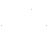# Sifter: Scalable Sampling for Distributed Tracing

written in

Distributed tracing can be ridiculously expensive if you try to trace a hundred percent of requests. A common technique to reduce costs is to sample only a small portion of the traffic. But naive sampling techniques like uniform sampling will inevitably capture more common-case executions and might miss the more interesting edge cases. Instead, Sifter’s approach is to bias sampling decisions towards outliers and anomalous traces. This way, anomalous traces have a higher chance of being sampled, and the more uninteresting traces are discarded.

## How it works

Sifter uses the incoming stream of traces to builds a model that approximates the system’s common-case behavior. To make a sampling decision, it checks how well the model matches the incoming trace. Sampling is biased toward traces that are not well approximated (outliers and anomalous traces).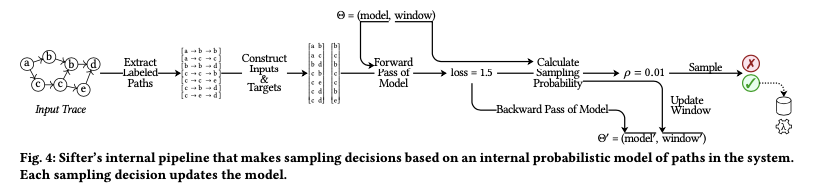### The model

Sifter uses a 2-layer neural network that models the probability of an event occurring given its immediate predecessors and successors. To create this model, they first extract all N-length paths for each trace.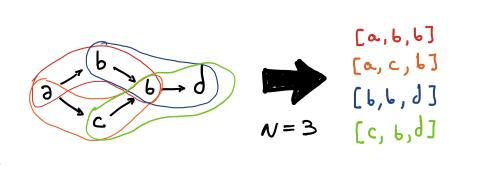For a given path (p_0, …, p_N), the neural network predicts the probability of p_N/2_ given its surrounding events p_0, … , p_N (excluding p_N/2_). The model gives better predictions for paths that are seeing more frequently in the input dataset.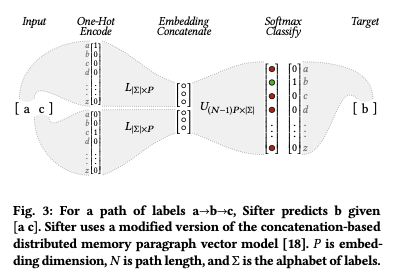### Sampling

To make a sampling decision, Sifter first extracts all N-length paths from traces and feeds that into the model. It then uses the output to calculate the prediction’s loss, that is the difference between the predicted and actual events. The higher the loss, the more “interesting” the trace is.

The loss is then weighted against the loss of the k most recent traces.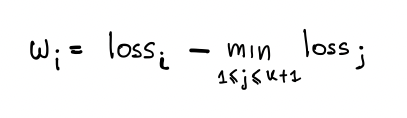If all traces have the same loss, then the trace is sampled uniformly at random with probability α. Otherwise, the probability is calculated using this formula: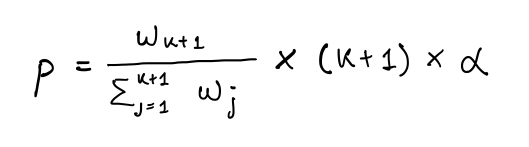Traces with the lowest loss will have a sampling probability of zero. Traces with the highest loss will have the highest sampling probability.

### Updating the model

Sifter does a gradient descent back-propagation pass on the model for every trace seen to keep the model up-to-date. It also adds the trace’s loss into the window of k most recent traces and pops the oldest value. Note that this happens on every trace regardless of whether it is sampled or not.

## Sifter’s Properties

The following list is a summary of Sifter’s design choices and their consequences:

• Sifter’s operates online over a continuous stream of traces. The overhead added by sampling is in the order of milliseconds. This latency is affected only by the trace size and the number of unique events in a trace. It is independent of the workload volume and the number of previously sampled traces.
• Sifter is not feature-based. The model used to identify interesting traces does not require any feature engineering from developers. As a consequence, Sifter’s approach is not limited to what developers consider interesting a priori. Instead, interesting features on the sampled traces can be discovered rather than engineered.
• As a corollary to the previous point, Sifter can automatically adapt to changes in traffic over time. The model is updated with every incoming trace, and the sampling probability is adjusted as the workload distribution changes.
• The amount of memory needed to keep Sifter’s model is a function of N the path size, E the label vocabulary, and k the number of most recently seen traces we consider. The total size is constant with respect to the workload volume and the number of sampling traces.
• Sifter does not require any pre-training or manual configuration before operation. On bootstrap, it’ll start making random sampling decisions while learning the model’s system behavior with every new trace.
• Sifter’s model converts traces into a directed acyclic graph of labels based on event origin, i.e. source file and line number. It does not consider other span features like timing, tags, annotations, etc.